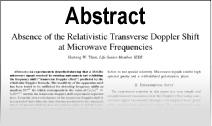Enter the content which will be displayed in sticky bar

# AbstractOrigin and Concept of Relativity, Part 1

Year: 1965
Keywords: Relativity

\"As far back as 1895, Poincar?, the innovator, had conjectured that it is impossible to detect absolute motion. In 1900 he introduced \'The principle of relative motion\' which he later called by the equivalent terms \'The law of relativity\' and \'The principle of relativity\' in his book Science and Hypothesis published in 1902. He further asserted in this book that there is no absolute time and that we have no intuition of the \'simultaneity\' of two \'events\' [mark the words] occurring at two different places. In a lecture given in 1904, Poincar? reiterated the principle of relativity, described the method of synchronization of clocks with light signals, urged a more satisfactory theory of the electrodynamics of moving bodies based on Lorentz\'s ideas and predicted a new mechanics characterized by the rule that the velocity of light cannot be surpassed. This was followed in June 1905 by a mathematical paper entitled \'Sur la dynamique de 1\'electron\', in which the connection between relativity (impossibility of detecting absolute motion) and the Lorentz transformation, given by Lorentz a year earlier, was recognized. [fn. Gravitational waves with velocity c and the velocity addition formula should be included (Keswani 1966).]

\"In point of fact, therefore, Poincar? was not only the first to enunciate the principle, but he also discovered in Lorentz\'s work the necessary mathematical formulation of the principle. All this happened before Einstein\'s paper appeared.\" [Keswani 1965]